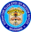Trending ▼   ResFinder# ICSE Prelims 2017 : 100+ super programs

8 pages, 131 questions, 96 questions with responses, 159 total responses,10Sai S Kalyan Kuriakose Elias English Medium School (KEEMS), Mannanam, Kottayam
+Fave Message
 Home > ved0_ssk_respaper_pongala >   F Also featured on: Group Page icseFormatting page ...

Write a program for the following, along with variable descriptions/mnemonics/comments 1. Check whether a number is prime-A number with only 1 and itself as its factors e.g.5 2. Check whether a number is composite-A number with factor(s) other than 1 and itself e.g. 6 3. Check whether a number is perfect-Equal to sum of factors other than itself e.g. 6 4. Check whether a number is special-Equal to the sum of factorials of its digits e.g.145 5. Check whether a number is Armstrong-Equal to the sum of cubes of its digits e.g.135 6. Check whether a number is odious-Contains odd number of 1s in binary form e.g. 5 7. Check whether a number is duck-Contains zeroes in it, not in the beginning e.g. 302 8. Check whether a number is disarium-Equal to the sum of digits raised to their positions from left e.g. 135 9. Check whether a number is IMEI-A 15 digit number which is divisible by the sum of last digit and twice the other 14 digits. e.g. 490154203237518 10. Check whether a number is twisted prime-a prime number whose reverse is also a prime number e.g. 13 11. Check whether a number is palindrome-Same as its reverse e.g.121 12. Check whether a number is automorphic-Contained in the last digits of its square e.g. 25 in 625 13. Check whether 2 numbers are amicable-Two numbers are amicable if the sum of factors of 1st number is equal to the 2nd and vice versa. 14. Check whether 2 numbers are coprimes-Are both prime and have only 1 as common factor. e.g. 23,29 15. Check whether a number is buzz number-Ends with 7 or divisible by 7 e.g. 67,177 16. Check whether a number is neon number-Equal to the sum of digits in its square e.g. 9, 9*9=81, 8+1=9 17. Check whether a number is valid ISBN- 10 digit no: such that sum of the 10 digits from left, multiplied by their respective positions, is divisible by 11 e.g. 14016014991+8+0+4+30+0+7+32+81+90=253, divisible by 11 18. Find the HCF and LCM of 2 numbers input by the user e.g. 15,25 HCF-5, LCM-75 19. Check whether a number is unique-a number whose digits are not repeated e.g. 12435 20. Check whether a number is happy number-The eventual sum of squares of digits should be 1 e.g. 28-22+82=68,62+82=100, 12+02+02=1 21. Check whether a number is a perfect cube e.g. 125=53 22. Check whether a number is a perfect square e.g. 25=52 23. Check whether a number is a niven number-divisible by sum of its digits e.g.126 24. Accept user s choice and display binary form of a decimal number for choice 1, decimal form of a binary number for choice 2. 25. Convert time input in 24 hour clock (military time) to time in 12 hour clock e.g. I/P-15:30 O/P-03:30 pm, I/P-07:24 O/P-07:24 am 26. Find the highest prime factor of a number e.g. I/P-12 , O/P-3Formatting page ...

Top Contributors
to this ResPaper.(24)PHYSICIST_Mainak(23)RaBh(18)Saransh Srivastava(13)Formatting page ...Formatting page ...Formatting page ...Formatting page ...Formatting page ...Formatting page ...